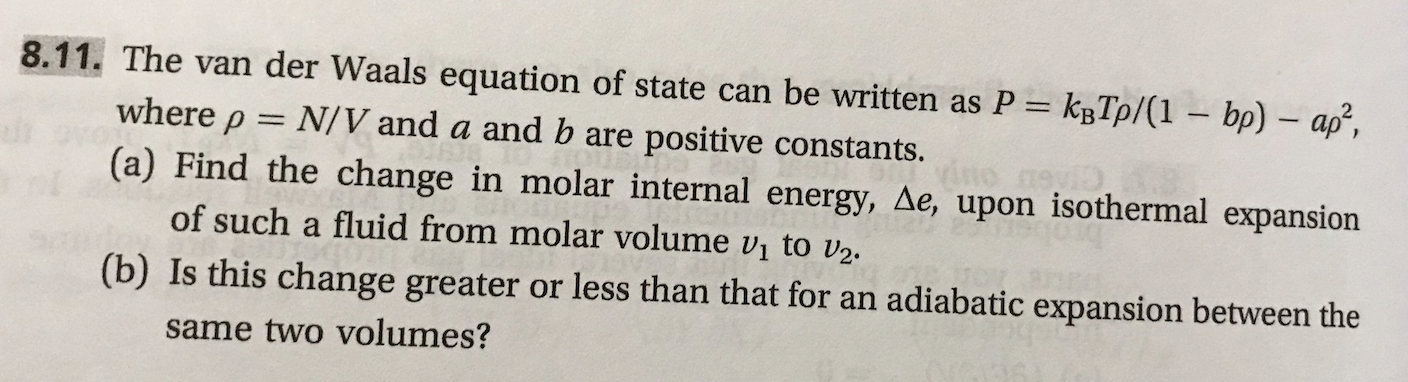# 8.11. The van der Waals equation of state can be written as P kTp/(1 bp) ap,where ρN/V and a and b are positive constants.(e) Find the change in molar internal energy, ae, upon lsothermal expansion(b) Is this change greater or less than that for an adiabatic expansion between theof such a fluid from molar volume v1 to U2,same two volumes?

Question
90 views

This problem is (8.11) from a book  "Thermodynamics and Statistical Mechanics An Integrated Approach by M. Scott Shell"help_outlineImage Transcriptionclose8.11. The van der Waals equation of state can be written as P kTp/(1 bp) ap, where ρ N/V and a and b are positive constants. (e) Find the change in molar internal energy, ae, upon lsothermal expansion (b) Is this change greater or less than that for an adiabatic expansion between the of such a fluid from molar volume v1 to U2, same two volumes? fullscreen
check_circle

Step 1

Part (a)

For van der Waals equation of states, the equation used for internal energy, E is written below. Divide this equation by N to get an equation for molar internal energy as:

Step 2

Now, for isothermal expansion from molar volume v1 to v2, change in the molar internal energy becomes:

Step 3

Part (b)

For an adiabatic expansion, Q=0. Thus, from first law of thermodynamics,

dE = dQ + dW

dE = 0 – PdV

In this equation, substitute the v...

### Want to see the full answer?

See Solution

#### Want to see this answer and more?

Solutions are written by subject experts who are available 24/7. Questions are typically answered within 1 hour.*

See Solution
*Response times may vary by subject and question.
Tagged in

### Chemical Engineering